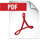# Implementation of interleaved mode using pseudo3D experiment

• Summary

## NM190017E

Implementing a series of NMR experiments in interleaved mode, ensures that all the experiments are conducted under identical conditions.
Delta software is fully transparent in how implementation is carried out and offers different options for the user to choose from. The "interleave" acquisition parameter provides an easy and fast solution. In the case of a series of 2D experiments, it is possible to implement interleaving through a pseudo3D experiment.

## Interleaving via a pseudo3D experiment

Any 3D NMR experiment is essentially a series of 1D experiments (t3, direct dimension) obtained by incrementing two variables, t1 and t2 (indirect dimensions). The variable of the indirect dimension defined as y is incrementing first. So if t1 has been assigned to y dimension and t2 to z dimension, t1 is incrementing first before t2. Suppose that in a 2D experiment with t1 in the y indirect dimension, t1 is reassigned to z dimension and an array of two points is assigned as y dimension. Assume that each one of these two points represent a different experiment. The result would be a pseudo3D experiment where the two experiments are carried out in an interleave mode. Interleaving occurs at the level of original t1 loop (t2 for the pseudo3D). The two experiments would complete simultaneously and would be stored in one single pseudo3D file.

## An implementation example

A jxp file for the IPAP experiment (1H-15N HSQC type) was modified in order to obtain a pseudo3D experiment with y dimension assigned to an array of two points representing the IP and AP experiment. Since y dimension is incremented before the z dimension (15N ), the two experiments IP and AP are collected in interleaved mode. At each point on z axis (15N ), IP and AP FIDs are collected sequentially. Thus, interleaving takes place at the level of t1 loop (t2 for the pseudo3D). Experiment was tested on a sample of u-15N labeled Ubiquitin in 10%D2O.Fig 1: For each point Z (15N dimension), IP (Y=1) and AP (Y=2) experiments are executed sequentially. In other words, the interleave loop is outer to scan loop and inner to 15N loop.Fig 2: Obtained pseudo3D data opened with processor window.Fig 3: The XZ plane at Y=1 is the IP spectrum (left) and the XZ plane at Y=2 is the AP spectrum (right)Fig 4: The two planes corresponding to IP and AP experiments can be saved separately. Then the two files can be combined in order to obtain the upfield or the downfield doublet component.Fig 5: Result of the addition of IP and AP spectra. Only the downfield doublet component is observed.

console：JNM-ECZ400S+ROYALPROBE™

If you want to see a printed version, please click this PDF file.PDF 613.2 KB